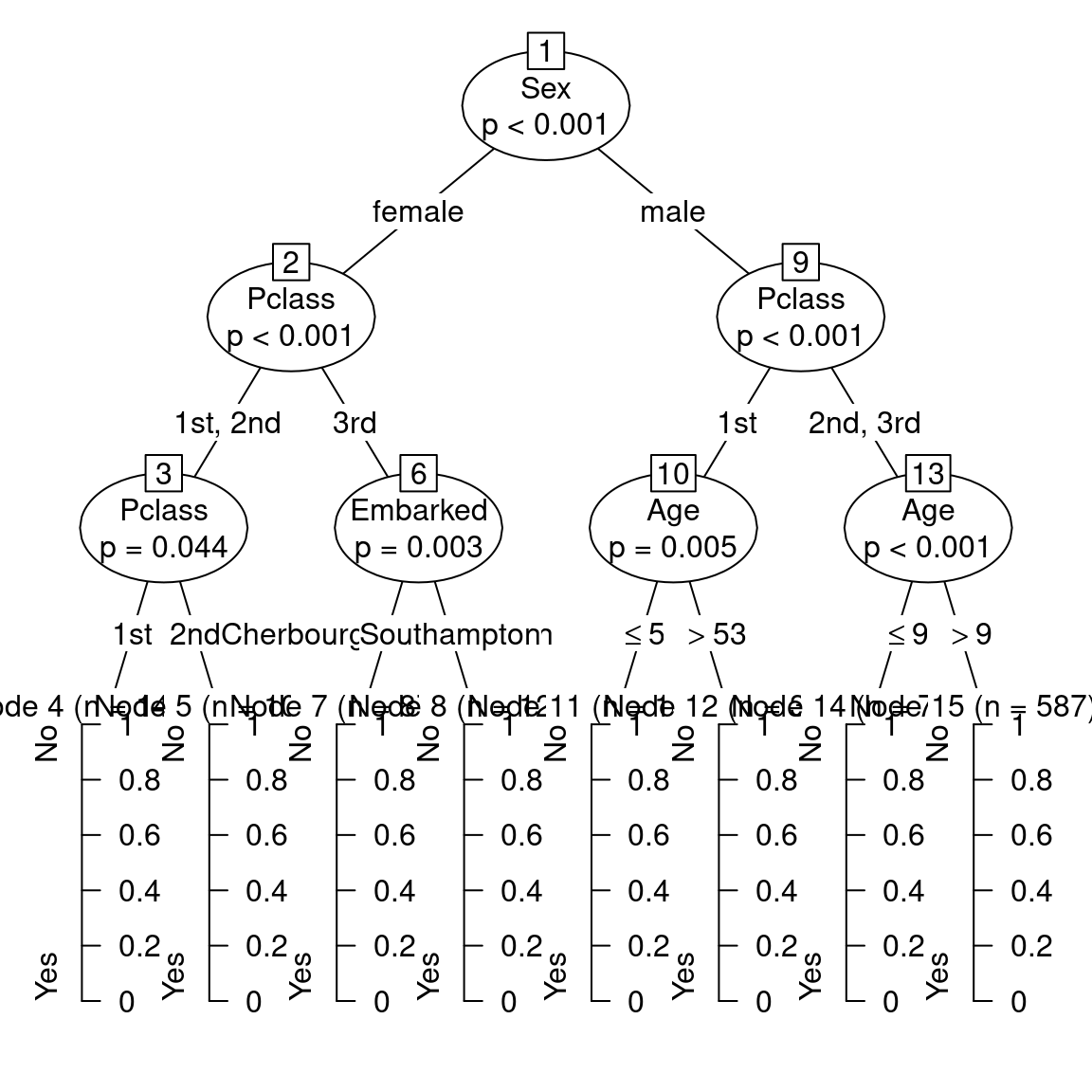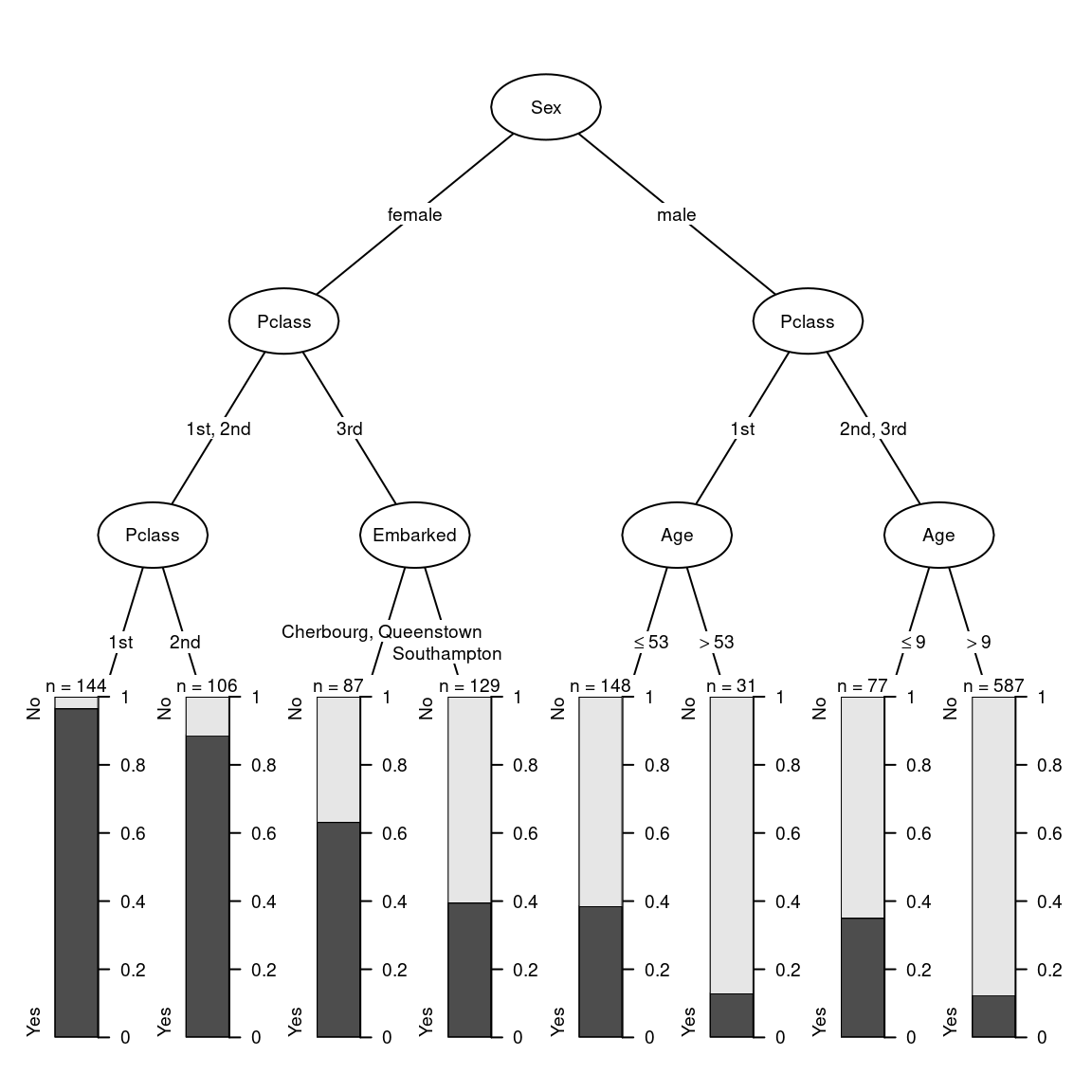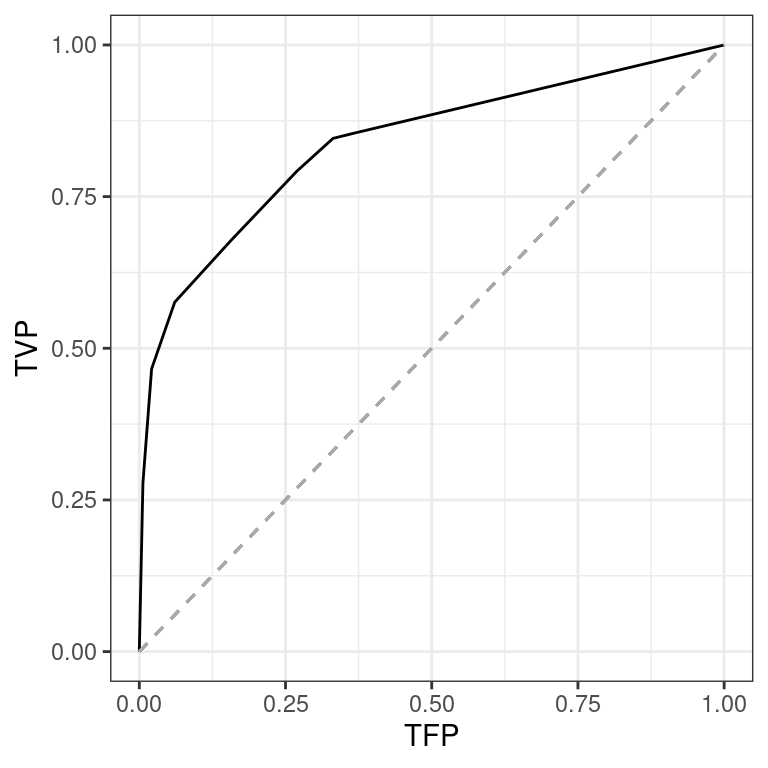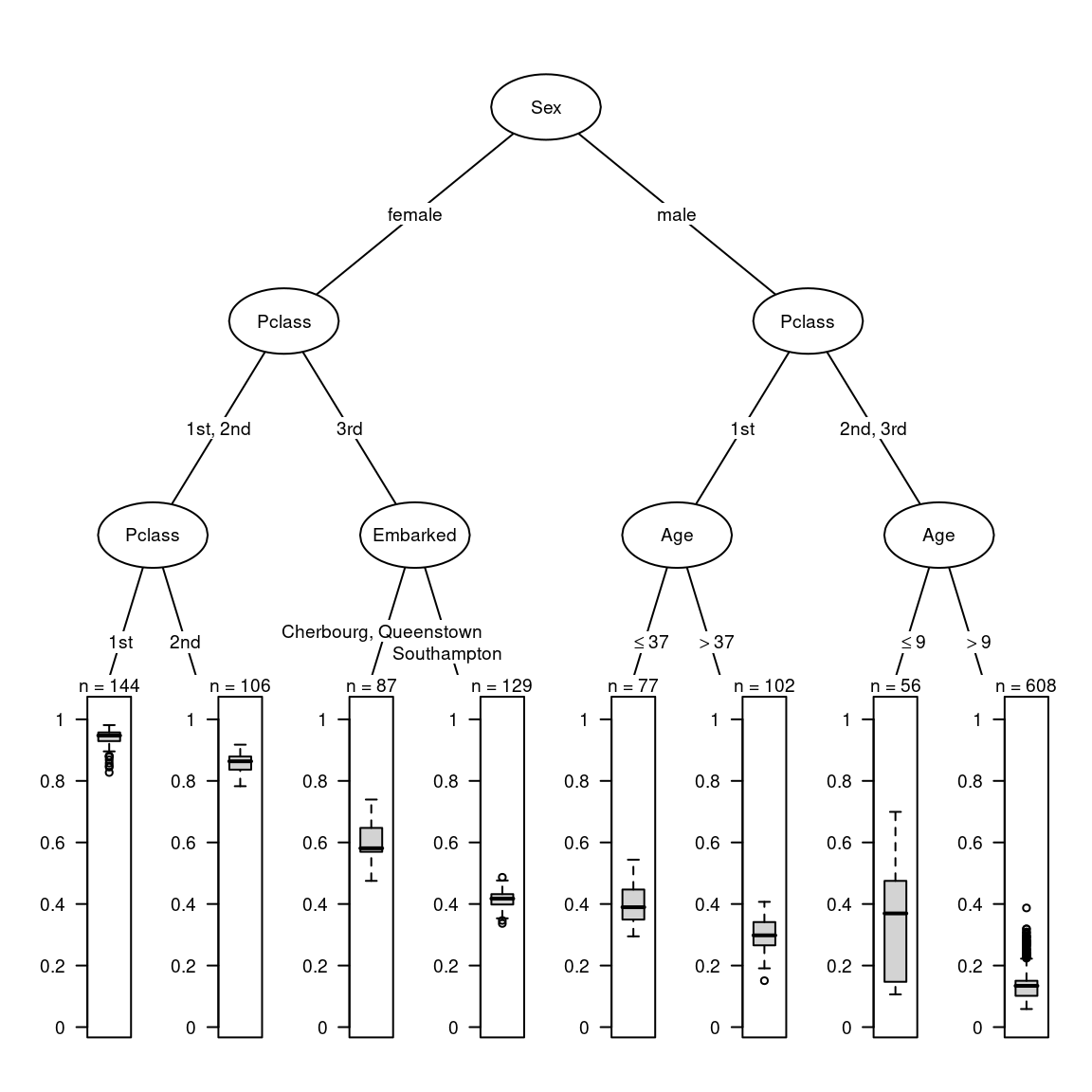# [en] An example of recursive partitioning with Titanic data

## First steps

First, the necessary packages are loaded into memory.

``````library(tidyverse)  # data management
library(caret)  # confusion matrix
library(party)  # conditional inference random forests and trees
library(partykit)  # conditional inference trees
library(pROC)  # ROC curves
library(measures)  # performance measures
library(varImp)  # variable importance
library(pdp)  # partial dependence
library(vip)  # measure of interactions
library(moreparty)  # surrogate trees, accumulated local effects, etc.
library(RColorBrewer)  # color palettes
library(GDAtools)  # bivariate analysis``````

Now, we then import `titanic` data set from `moreparty`.

``````data(titanic)
str(titanic)``````
``````spec_tbl_df [1,309 × 5] (S3: spec_tbl_df/tbl_df/tbl/data.frame)
\$ Survived: Factor w/ 2 levels "No","Yes": 2 2 1 1 1 2 2 1 2 1 ...
\$ Sex     : Factor w/ 2 levels "female","male": 1 2 1 2 1 2 1 2 1 2 ...
\$ Pclass  : Factor w/ 3 levels "1st","2nd","3rd": 1 1 1 1 1 1 1 1 1 1 ...
\$ Age     : num [1:1309] 29 0.917 2 30 25 ...
\$ Embarked: Factor w/ 3 levels "Cherbourg","Queenstown",..: 3 3 3 3 3 3 3 3 3 1 ...``````

We have 1309 cases, one categorical explained variable, `Survived`, which codes whether or not an individual survived the shipwreck, and four explanatory variables (three categorical and one continuous): gender, age, passenger class, and port of embarkation. The distribution of the variables is examined.

``summary(titanic)``
`````` Survived      Sex      Pclass         Age                 Embarked
No :809   female:466   1st:323   Min.   : 0.1667   Cherbourg  :270
Yes:500   male  :843   2nd:277   1st Qu.:21.0000   Queenstown :123
3rd:709   Median :28.0000   Southampton:914
Mean   :29.8811   NA's       :  2
3rd Qu.:39.0000
Max.   :80.0000
NA's   :263                        ``````

The distribution of the explained variable is not balanced, as survival is largely in the minority. In addition, some explanatory variables have missing values, in particular `Age`.

We examine the bivariate statistical relationships between the variables.

``BivariateAssoc(titanic\$Survived, titanic[,-1])``
``````\$YX
variable measure assoc p.value   criterion
1      Sex  cramer 0.527 0.00000 0.000000000
2   Pclass  cramer 0.313 0.00000 0.000000000
3 Embarked  cramer 0.184 0.00000 0.000000001
4      Age    eta2 0.002 0.26069 0.302040642

\$XX
variable1 variable2 measure assoc p.value    criterion
1    Pclass       Age    eta2 0.170 0.00000 0.0000000000
2    Pclass  Embarked  cramer 0.280 0.00000 0.0000000000
3       Sex    Pclass  cramer 0.125 0.00004 0.0000378611
4       Sex  Embarked  cramer 0.122 0.00006 0.0000563134
5       Age  Embarked    eta2 0.006 0.01789 0.0180491352
6       Sex       Age    eta2 0.003 0.03964 0.0404512887``````

Survival is primarily associated with gender, secondarily with the passenger class. The explanatory variables are weakly related to each other.

``catdesc(titanic\$Survived, titanic[,-1], min.phi=0.1, robust=FALSE)``
``````\$variables
variable    measure association permutation.pvalue
1      Sex Cramer's V       0.529                 NA
2   Pclass Cramer's V       0.313                 NA
3 Embarked Cramer's V       0.184                 NA
4      Age       Eta2       0.003                 NA

\$bylevel
\$bylevel\$No
\$bylevel\$No\$categories
categories pct.ycat.in.xcat pct.xcat.in.ycat pct.xcat.global
2              Sex.male            0.809            0.843           0.644
3            Pclass.3rd            0.745            0.653           0.542
6  Embarked.Southampton            0.667            0.754           0.699
8    Embarked.Cherbourg            0.444            0.148           0.207
9            Pclass.1st            0.381            0.152           0.247
11           Sex.female            0.273            0.157           0.356
phi
2   0.529
3   0.283
6   0.155
8  -0.182
9  -0.279
11 -0.529

\$bylevel\$No\$continuous.var
variables mean.x.in.ycat mean.x.global sd.x.in.ycat sd.x.global   cor
1       Age             NA            NA           NA          NA 0.056

\$bylevel\$Yes
\$bylevel\$Yes\$categories
categories pct.ycat.in.xcat pct.xcat.in.ycat pct.xcat.global
1            Sex.female            0.727            0.678           0.356
4            Pclass.1st            0.619            0.400           0.247
5    Embarked.Cherbourg            0.556            0.301           0.207
7  Embarked.Southampton            0.333            0.610           0.699
10           Pclass.3rd            0.255            0.362           0.542
12             Sex.male            0.191            0.322           0.644
phi
1   0.529
4   0.279
5   0.182
7  -0.155
10 -0.283
12 -0.529

\$bylevel\$Yes\$continuous.var
variables mean.x.in.ycat mean.x.global sd.x.in.ycat sd.x.global    cor
2       Age             NA            NA           NA          NA -0.056``````

Women, first class passengers and those who boarded at Cherbourg are over-represented among the survivors. Men, 3rd class passengers and those who boarded at Southampton are over-represented among the non-survivors.

Random forests imply a share of randomness (via resampling and drawing of splitting variables), as well as some interpretation tools (via variable permutations). From one program run to the next, the results may therefore differ slightly. If you wish to obtain the same results systematically and to ensure reproducibility, use the `set.seed` function.

``set.seed(1912)``

## Classification tree

In order to build a classification tree with CTree conditional inference algorithm, we use `partykit` package, which allows more flexibility than `party` package, in particular to deal with missing values.

The tree can be displayed in textual or graphical form.

``````arbre <- partykit::ctree(Survived~., data=titanic, control=partykit::ctree_control(minbucket=30, maxsurrogate=Inf, maxdepth=3))

print(arbre)``````
``````
Model formula:
Survived ~ Sex + Pclass + Age + Embarked

Fitted party:
 root
|    Sex in female
|   |    Pclass in 1st, 2nd
|   |   |    Pclass in 1st: Yes (n = 144, err = 3.5%)
|   |   |    Pclass in 2nd: Yes (n = 106, err = 11.3%)
|   |    Pclass in 3rd
|   |   |    Embarked in Cherbourg, Queenstown: Yes (n = 87, err = 36.8%)
|   |   |    Embarked in Southampton: No (n = 129, err = 39.5%)
|    Sex in male
|   |    Pclass in 1st
|   |   |    Age <= 53: No (n = 148, err = 38.5%)
|   |   |    Age > 53: No (n = 31, err = 12.9%)
|   |    Pclass in 2nd, 3rd
|   |   |    Age <= 9: No (n = 77, err = 35.1%)
|   |   |    Age > 9: No (n = 587, err = 12.4%)

Number of inner nodes:    7
Number of terminal nodes: 8``````
``plot(arbre)```Sex` is the first splitting variable, `Pclass` is the second and all the explanatory variables are used in the tree.

The proportion of survivors varies greatly from one terminal node to another.

``nodeapply(as.simpleparty(arbre), ids = nodeids(arbre, terminal = TRUE), FUN = function(x) round(prop.table(info_node(x)\$distribution),3))``
``````\$`4`
No   Yes
0.035 0.965

\$`5`
No   Yes
0.113 0.887

\$`7`
No   Yes
0.368 0.632

\$`8`
No   Yes
0.605 0.395

\$`11`
No   Yes
0.615 0.385

\$`12`
No   Yes
0.871 0.129

\$`14`
No   Yes
0.649 0.351

\$`15`
No   Yes
0.876 0.124 ``````

Thus, 96.5% of women travelling in 1st class survive, compared with only 12.4% of men over 9 years of age travelling in 2nd or 3rd class.

The graphical representation can be parameterized to obtain a simpler and more readable tree.

``plot(arbre, inner_panel=node_inner(arbre,id=FALSE,pval=FALSE), terminal_panel=node_barplot(arbre,id=FALSE), gp=gpar(cex=0.6), ep_args=list(justmin=15))``Note that the `ggparty` package graphically represents Ctree trees with the `ggplot2` grammar.

To measure the performance of the tree, the AUC is calculated, by comparing predicted and observed survival.

``````pred_arbre <- predict(arbre, type='prob')[,'Yes']

auc_arbre <- AUC(pred_arbre, titanic\$Survived, positive='Yes')
auc_arbre %>% round(3)``````
`` 0.838``

AUC is 0.838, which is a relatively high performance.

To plot the ROC curve of the model :

``````pROC::roc(titanic\$Survived, pred_arbre) %>%
ggroc(legacy.axes=TRUE) +
geom_segment(aes(x=0,xend=1,y=0,yend=1), color="darkgrey", linetype="dashed") +
theme_bw() +
xlab("TFP") +
ylab("TVP")``````Other performance measures are based on the confusion matrix.

``````ifelse(pred_arbre > .5, "Yes", "No") %>%
factor %>%
caret::confusionMatrix(titanic\$Survived, positive='Yes')``````
``````Confusion Matrix and Statistics

Reference
Prediction  No Yes
No  760 212
Yes  49 288

Accuracy : 0.8006
95% CI : (0.7779, 0.8219)
No Information Rate : 0.618
P-Value [Acc > NIR] : < 2.2e-16

Kappa : 0.5497

Mcnemar's Test P-Value : < 2.2e-16

Sensitivity : 0.5760
Specificity : 0.9394
Pos Pred Value : 0.8546
Neg Pred Value : 0.7819
Prevalence : 0.3820
Detection Rate : 0.2200
Detection Prevalence : 0.2574
Balanced Accuracy : 0.7577

'Positive' Class : Yes
``````

The `GetSplitStats` function allows to examine the result of the competition between covariates in the choice of splitting variables. If, for a given node, the splitting variable is significantly more associated with the explained variable than the other explanatory variables, we can think that this split is stable.

``GetSplitStats(arbre)``
``````\$`1`
statistic      p.value     criterion        ratio
Sex      365.607431 6.771232e-81 -6.771232e-81 1.000000e+00
Pclass   127.761479 7.227818e-28 -7.227818e-28 1.067430e+53
Embarked  44.207893 1.005629e-09 -1.005629e-09 1.485150e+71
Age        3.220314 2.606920e-01 -3.020406e-01 4.460645e+79

\$`2`
statistic      p.value     criterion        ratio
Pclass   115.454414 2.549845e-25 -2.549845e-25 1.000000e+00
Embarked  24.336500 1.557812e-05 -1.557824e-05 6.109486e+19
Age        7.070397 2.332660e-02 -2.360298e-02 9.256632e+22

\$`3`
statistic    p.value   criterion    ratio
Pclass   5.9107118 0.04447125 -0.04549043  1.00000
Embarked 4.2482494 0.31745308 -0.38192401  8.39570
Age      0.2003411 0.95873811 -3.18781595 70.07663

\$`6`
statistic     p.value   criterion    ratio
Embarked 12.759466 0.003388277 -0.00339403   1.0000
Age       1.724815 0.342399641 -0.41915789 123.4986

\$`9`
statistic      p.value     criterion     ratio
Pclass    32.99374 2.054102e-07 -2.054103e-07     1.000
Embarked  17.71078 4.277725e-04 -4.278640e-04  2082.973
Age       10.83849 2.979392e-03 -2.983839e-03 14526.240

\$`10`
statistic    p.value    criterion    ratio
Age       9.079224 0.00516391 -0.005177289   1.0000
Embarked  2.193883 0.55629854 -0.812603321 156.9554

\$`13`
statistic      p.value     criterion     ratio
Age      25.40589207 1.393491e-06 -1.393492e-06       1.0
Embarked  5.27150955 1.999551e-01 -2.230874e-01  160092.3
Pclass    0.03486032 9.967509e-01 -5.729373e+00 4111521.7``````

In this case, for each of the nodes, the result of the competition is final (see `criterion`). The tree therefore appears to be stable.

## Random forest

Then we build a random forest with conditional inference algorithm, `mtry`=2 and `ntree`=500.

``foret <- party::cforest(Survived~., data=titanic, controls=party::cforest_unbiased(mtry=2,ntree=500))``

To compare the performance of the forest to that of the tree, the predictions and then the AUC are calculated.

``````pred_foret <- predict(foret, type='prob') %>%
do.call('rbind.data.frame',.) %>%
select(2) %>%
unlist

auc_foret <- AUC(pred_foret, titanic\$Survived, positive='Yes')
auc_foret %>% round(3)``````
`` 0.879``

The performance of the forest is 0.879, therefore slightly better than that of the tree.

The `OOB=TRUE` option allows predictions to be made from out-of-bag observations, thus avoiding optimism bias.

``````pred_oob <- predict(foret, type='prob', OOB=TRUE) %>%
do.call('rbind.data.frame',.) %>%
select(2) %>%
unlist

auc_oob <- AUC(pred_oob, titanic\$Survived, positive='Yes')
auc_oob %>% round(3)``````
`` 0.847``

Calculated in this way, the performance is indeed slightly lower (0.847).

## Surrogate tree

The so-called “surrogate tree” can be a way to synthesize a complex model.

``````surro <- SurrogateTree(foret, maxdepth=3)

surro\$r.squared %>% round(3)``````
`` 0.96``
``plot(surro\$tree, inner_panel=node_inner(surro\$tree,id=FALSE,pval=FALSE), terminal_panel=node_boxplot(surro\$tree,id=FALSE), gp=gpar(cex=0.6), ep_args=list(justmin=15))``The surrogate tree reproduces here very faithfully the predictions of the random forest (R2 = 0.96). It is also very similar to the initial classification tree.

## Variable importance

To go further in the interpretation of the results, permutation variable importance is calculated (using AUC as a performance measure). Since the explanatory variables have little correlation with each other, it is not necessary to use the “conditional permutation scheme” (available with the `conditional=TRUE` option in the `varImpAUC` function).

``````importance <- -varImpAUC(foret)
importance %>% round(3)``````
``````     Sex   Pclass      Age Embarked
-0.213   -0.066   -0.026   -0.008 ``````
``ggVarImp(-importance)``